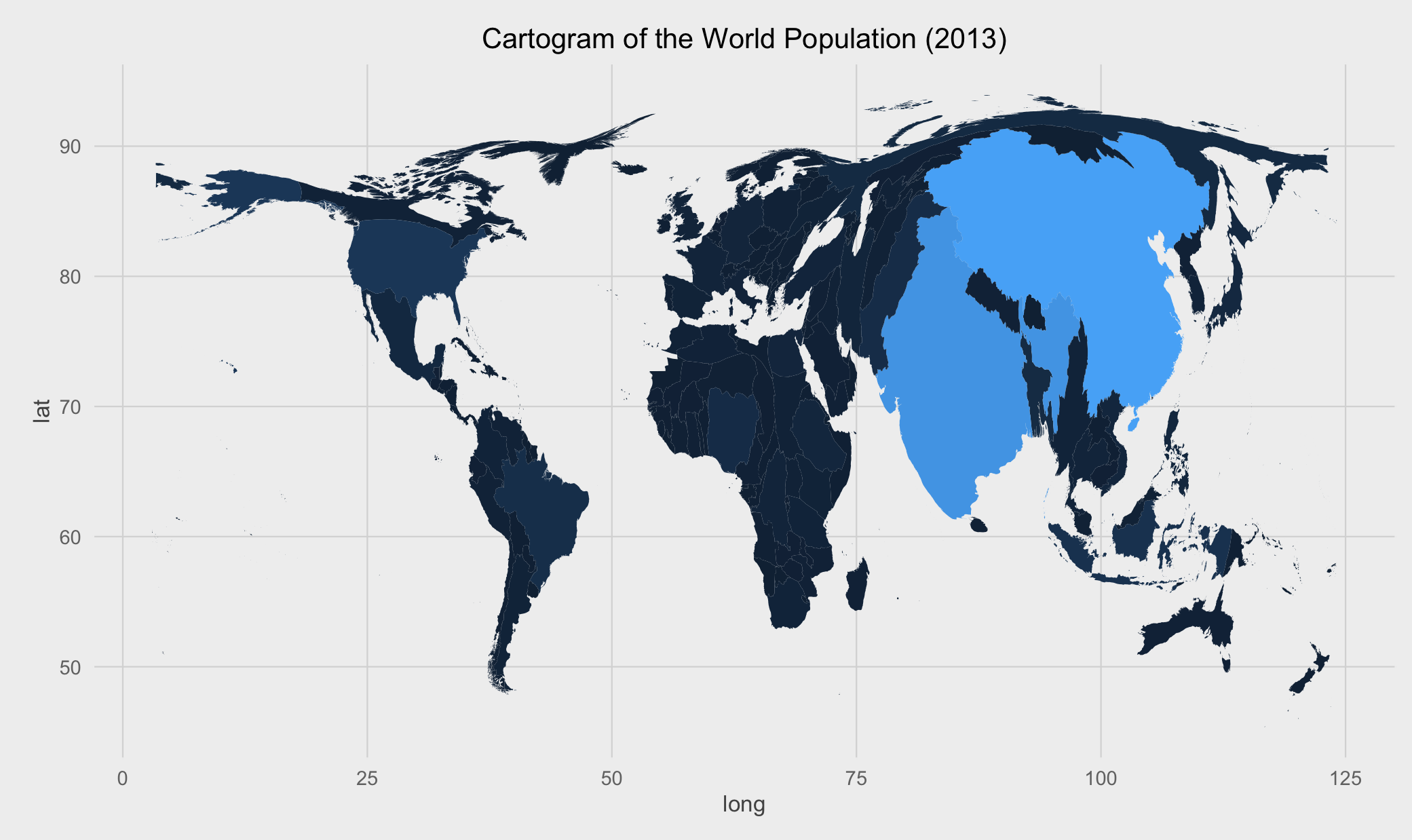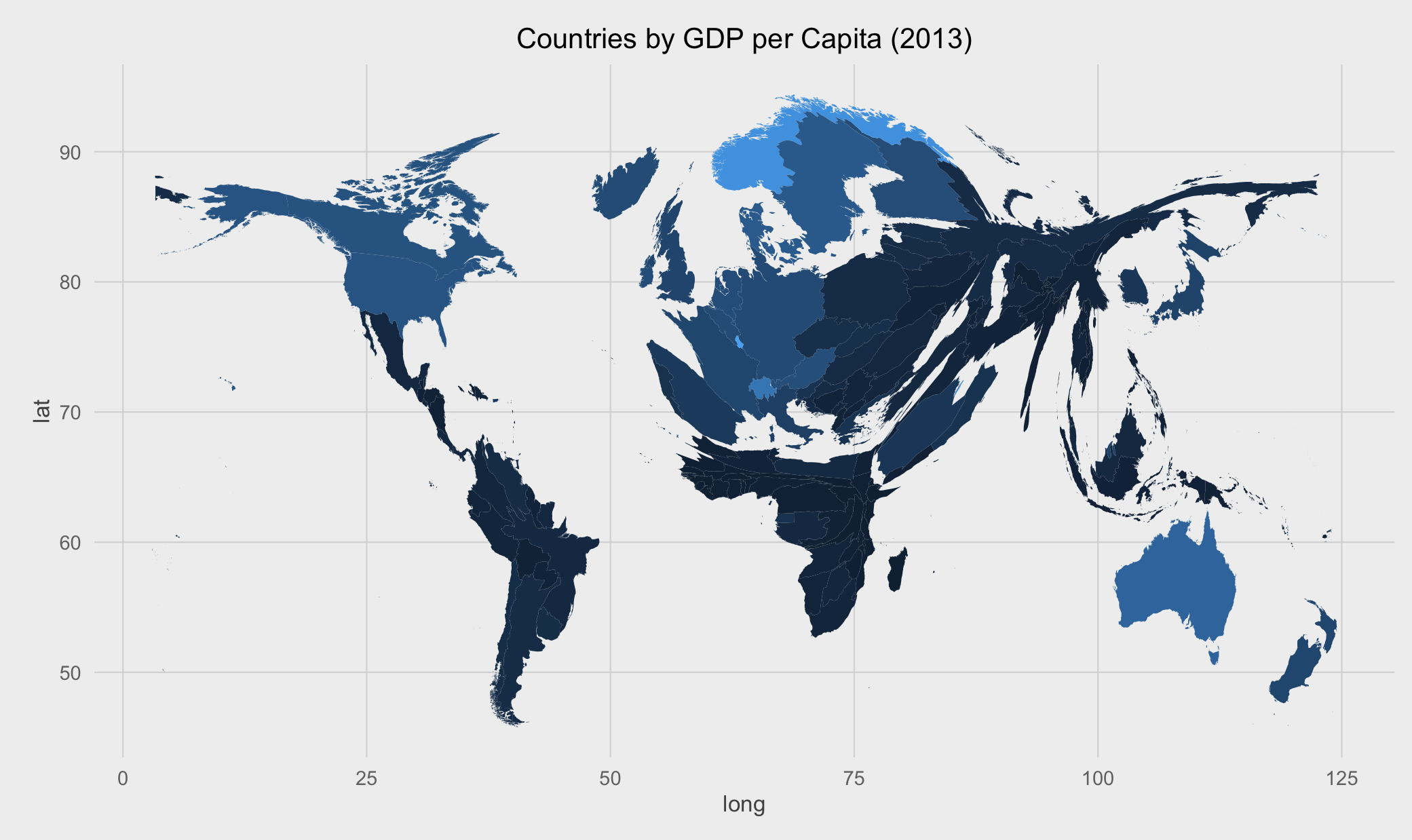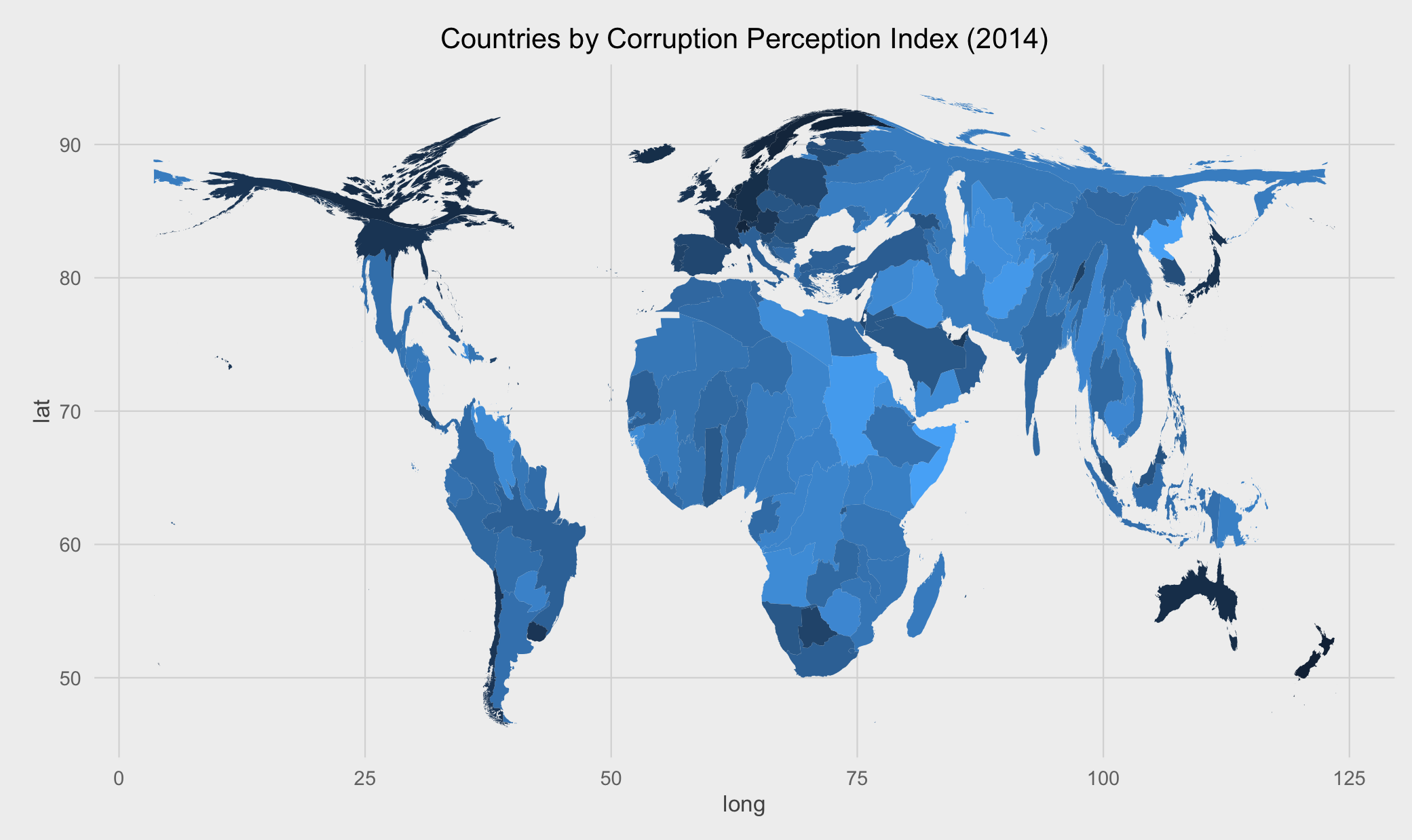A cartogram is a thematic map where a certain mapping variable (e.g., population or economic indicator) of a geographic region is substituted for its physical (land) area. The spatial geometry of the map is then proportionately distorted in order to reflect the information conveyed by the thematic variable. Cartograms are thus visually intuitive and revealing to the presentation of such information.

There exist a few algorithms to compute cartograms given the land densities and thematic variables. One of them is the diffusion-based method proposed by Gatsner and Newman, in which the authors also provide a neat C program to compute the point-wise cartogram transformations. Check out some of the very revealing maps extracted from their book Atlas of the Real World.

This post demonstrates how to compute and plot cartograms using R and given input shapefiles by interfacing with the above-mentioned C program via two packages: Rcartogram and getcartr. I was first motivated by this post, in which the author did not find an easy way to make cartograms using R and resorted to using the off-the-shelf tool ScapeToad. In this blog post, I will show how to plot the cartogram of the world’s population by country in 2013, whose data can be downloaded here.

All the source code in this tutorial can be downloaded from this GitHub repo.

### Preparations

First, make sure you have the two packages FFTW3 and “fftw” for R, which perform fast Fourier transform, properly installed. For Linux and macOS, do the following (courtesy of this article):

• Extract to a directory and `cd` there.
• On the Terminal, type: `./configure --enable-shared`
• Then compile the package: `make`, and install it: `sudo make install`

If nothing goes wrong, the library should be correctly installed. Now, download fftw to some directory. In that directory, type: `sudo R CMD INSTALL fftw_1.0-3.tar.gz`, where `fftw_1.0-3.tar.gz` is the name of the downloaded file. This would compile and install the R package.

The next steps are to be performed in an R environment (e.g., RStudio). We are now going to install two R packages from GitHub: Rcartogram and getcartr. (Thus, make sure you have devtools package installed.) The former bundles the compiled C binaries with some neat R functions to interface with them. Whereas, the latter provides a means to interface with `Rcartogram` and the C library through which the input shapefile and its carto-transformed polygons can be effectively plotted.

Finally, make sure you have ggplot2maptools, and rgeos properly installed in R for geospatial visualization and data manipulations. (The last two are required for `getcartr`, anyways.)

First, load all the necessary packages at the beginning of your script:

Then, read the shapefile that contains the map that you wish to plot. In this case, it is the world map with country borders. The corresponding shapefile can be downloaded from here.

Next, read the data frame that contains the thematic variable of interest. In this example, it is the world’s population by country in 2013. This means the shape and size of each country (and respective boundaries) will be transformed and distorted proportionally to its population (and land area).

### Combining data and plotting the cartogram

In this last step, we will first join the two datasets `world` and `smaller.data` by their common field: `ISO3` and `Country.Code`, respectively. We then compute the cartogram transformations of all the density points that make up the areas of all the countries according to their populations using the `quick.carto` function. (ISO3 code is a 3-letter representation of all the countries in the world.)

In order to plot the resulted cartogram, we need to convert `world.carto` into a plottable data frame. The data frame will then be merged with the input shapefile using their shared field `Country.Code`.

Finally, this is the cartogram we wish to see:Compare this with the conventional thematic map and see the difference in how much more visual information a cartogram can convey!

Here are other two revealing cartograms: world countries by GDP per capita (2013) and the Corruption Perception Index (2014), respectively.